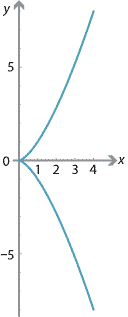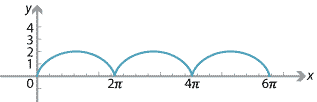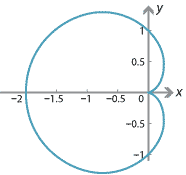### Finding gradients on a parametric curveConsider the parametric curve defined by \begin{align*} x &= t^2 \\ y &= t^3. \end{align*} We will find $$\dfrac{dy}{dx}$$ in terms of $$t$$ by using the chain rule and the result $\dfrac{dt}{dx} = \dfrac{1}{\dfrac{dx}{dt}}.$ We have $$\dfrac{dx}{dt} = 2t$$ and $$\dfrac{dy}{dt} = 3t^2$$. Hence, \begin{align*} \dfrac{dy}{dx} &= \dfrac{dy}{dt} \times \dfrac{dt}{dx} \\ &= \dfrac{3t^2}{2t} \\ &= \dfrac{3t}{2}. \end{align*}

#### Projectile motion

The motion of a particle projected at an angle $$\alpha$$ to the horizontal with an initial velocity of $$u$$ m/s can be described by the parametric equations \begin{align*} x &= u\cos(\alpha) t \\ y &= u\sin(\alpha) t - \dfrac{1}{2}gt^2, \end{align*} where $$g$$ is the acceleration due to gravity. A method for deriving these equations is given in the module Motion in a straight line. The velocities in the horizontal and vertical directions are $\dfrac{dx}{dt} = u\cos(\alpha) \qquad\text{and}\qquad \dfrac{dy}{dt} = u\sin(\alpha) - gt.$ Using the chain rule, we have $\dfrac{dy}{dx} = \dfrac{dy}{dt} \times \dfrac{dt}{dx} = \dfrac{u\sin(\alpha) - gt}{u\cos(\alpha)}.$ This gives the gradient of the path of the projectile in terms of $$t$$, for $$t \geq 0$$. This also allows us to find the angle of inclination of the path of the projectile at any time $$t$$. We use the fact that $\tan\theta = \dfrac{dy}{dx},$ where $$\theta$$ is the angle of inclination of the path to the horizontal. For example, if $$\alpha = \dfrac{\pi}{4}$$ and $$u = 10$$, then $\tan\theta = \dfrac{\dfrac{10}{\sqrt{2}}-gt}{\dfrac{10}{\sqrt{2}}} = 1 - \dfrac{\sqrt{2}gt}{10}.$

#### Cycloids

A cycloid is the curve traced by a point on the rim of a circular wheel as the wheel rolls along a straight line. For a wheel of radius 1, the parametric equations of the cycloid are

\begin{align*} x &= \theta-\sin\theta \\ y &= 1-\cos\theta. \end{align*}

The graph of $$y$$ against $$x$$ for $$0\leq \theta\leq 6\pi$$ is as follows.We can find the gradient at a point on the cycloid by using the chain rule. We have

$\dfrac{dx}{d\theta} = 1 - \cos\theta \qquad\text{and}\qquad \dfrac{dy}{d\theta} = \sin\theta.$

The chain rule gives

\begin{align*} \dfrac{dy}{dx} &= \dfrac{dy}{d\theta} \times \dfrac{d\theta}{dx} \\ &= \dfrac{\sin \theta}{1 - \cos\theta} \\ &= \dfrac{2\sin \dfrac{\theta}{2} \cos\dfrac{\theta}{2}}{2\sin^2\dfrac{\theta}{2}} \\ &= \cot\dfrac{\theta}{2}. \end{align*}

Also,

\begin{align*} \dfrac{d^2y}{dx^2} &= \dfrac{d}{dx} \Bigl(\dfrac{dy}{dx} \Bigr) \\ &= \dfrac{d}{dx} \Bigl(\cot\dfrac{\theta}{2}\Bigr) \\ &= \dfrac{d}{d\theta} \Bigl(\cot\dfrac{\theta}{2}\Bigr) \times \dfrac{d\theta}{dx} \\ &= -\dfrac{1}{4\sin^4(\dfrac{\theta}{2})}. \end{align*}
###### Notes.
• There are stationary points where $$\cot\dfrac{\theta}{2} = 0$$, which is equivalent to $$\cos\dfrac{\theta}{2} = 0$$. This occurs when $$\theta$$ is an odd multiple of $$\pi$$, that is, when $$\theta \in \{\dots, -5\pi, -3\pi, -\pi, \pi, 3\pi, 5\pi, \dots\}$$. Note that $$\dfrac{d^2y}{dx^2} < 0$$ for these values of $$\theta$$, and so there are local maxima when $$\theta$$ is an odd multiple of $$\pi$$.
• The function is not differentiable when $$\theta$$ is even multiple of $$\pi$$. That is, these are critical points of the function.
• There are no points of inflexion.
• The $$x$$-intercepts occur when $$\cos\theta = 1$$, that is, when $$\theta$$ is an even multiple of $$\pi$$.

#### CardioidsA cardioid is the curve traced by a point on the perimeter of a circle that is rolling around a fixed circle of the same radius. The cardioid shown in the graph has parametric equations

\begin{align*} x &= (1-\cos t)\cos t \\ y &= (1-\cos t)\sin t. \end{align*}

It is plotted for $$t \in [0,2\pi]$$.

We can calculate the gradient of the cardioid for a particular value of $$t$$ as follows:

\begin{align*} \dfrac{dx}{dt} &= -\sin t + 2\sin t \cos t = \sin 2t - \sin t \\ \dfrac{dy}{dt} &= \cos t - \cos^2 t + \sin^2 t = \cos t - \cos 2t, \end{align*} and so $\dfrac{dy}{dx} = \dfrac{dy}{dt} \times \dfrac{dt}{dx} = \dfrac{\cos t - \cos 2t}{\sin 2t - \sin t}.$

The gradient is defined for $$\sin 2t - \sin t \neq 0$$. For $$t \in [0,2\pi]$$, this means that gradient is not defined for $$t = 0, \dfrac{\pi}{3}, \pi, \dfrac{5\pi}{3}, 2\pi$$. The corresponding $$x$$-values are $$0, \dfrac{1}{4}, -2, \dfrac{1}{4}, 0$$.

There are points of zero gradient when $$t = \dfrac{2\pi}{3}, \dfrac{4\pi}{3}$$, which corresponds to $$x = -\dfrac{3}{4}$$.

Next page - History and applications